Please keep in mind, that DALEXtra now support usage of mlr with dedicated `explain_h2o` function. It is recommended to use it.

# 1 Introduction

DALEX is designed to work with various black-box models like tree ensembles, linear models, neural networks etc. Unfortunately R packages that create such models are very inconsistent. Different tools use different interfaces to train, validate and use models.

In this vignette we will show explanations for models created with h2o package.

# 2 Regression use case - apartments data

``````library("DALEX")
library("h2o")
set.seed(123)``````

To illustrate applications of DALEX to regression problems we will use an artificial dataset apartments available in the DALEX package. Our goal is to predict the price per square meter of an apartment based on selected features such as construction year, surface, floor, number of rooms, district. It should be noted that four of these variables are continuous while the fifth one is a categorical one. Prices are given in Euro.

``````data(apartments)
``````##   m2.price construction.year surface floor no.rooms    district
## 1     5897              1953      25     3        1 Srodmiescie
## 2     1818              1992     143     9        5     Bielany
## 3     3643              1937      56     1        2       Praga
## 4     3517              1995      93     7        3      Ochota
## 5     3013              1992     144     6        5     Mokotow
## 6     5795              1926      61     6        2 Srodmiescie``````

# 3 Models

We create two regular H2O models: glm and gbm. To do this w need to first initialize h2o and we need to convert apartments to H2OFrame.

``h2o.init()``
``````##
## H2O is not running yet, starting it now...
##
## Note:  In case of errors look at the following log files:
##     C:\Users\szyna\AppData\Local\Temp\RtmpUVYHo0/h2o_szyna_started_from_r.out
##     C:\Users\szyna\AppData\Local\Temp\RtmpUVYHo0/h2o_szyna_started_from_r.err
##
##
## Starting H2O JVM and connecting: . Connection successful!
##
## R is connected to the H2O cluster:
##     H2O cluster uptime:         4 seconds 299 milliseconds
##     H2O data parsing timezone:  UTC
##     H2O cluster version:        3.24.0.5
##     H2O cluster version age:    4 months and 29 days !!!
##     H2O cluster name:           H2O_started_from_R_szyna_osn291
##     H2O cluster total nodes:    1
##     H2O cluster total memory:   1.97 GB
##     H2O cluster total cores:    4
##     H2O cluster allowed cores:  4
##     H2O cluster healthy:        TRUE
##     H2O Connection ip:          localhost
##     H2O Connection port:        54321
##     H2O Connection proxy:       NA
##     H2O Internal Security:      FALSE
##     H2O API Extensions:         Amazon S3, Algos, AutoML, Core V3, Core V4
##     R Version:                  R version 3.6.1 (2019-07-05)``````
``````## Warning in h2o.clusterInfo():
## Your H2O cluster version is too old (4 months and 29 days)!
``````h2o.no_progress()

apartments_hf <- as.h2o(apartments)

model_h2o_glm <- h2o.glm(y = "m2.price", training_frame = apartments_hf)
model_h2o_gbm <- h2o.gbm(y = "m2.price", training_frame = apartments_hf)``````

We also create third model by an H2O AutoML.

``model_h2o_automl <- h2o.automl(y = "m2.price", training_frame = apartments_hf, max_models = 10)``

# 4 The explain() function

The first step of using the DALEX package is to wrap-up the black-box model with meta-data that unifies model interfacing.

To create an explainer we use `explain()` function. Validation dataset for the models is `apartmentsTest` data from the `DALEX` package. For the models created by h2o package we have to provide custom predict function which takes two arguments: `model` and `newdata` and returns a numeric vector with predictions.

``````custom_predict <- function(model, newdata)  {
newdata_h2o <- as.h2o(newdata)
res <- as.data.frame(h2o.predict(model, newdata_h2o))
return(as.numeric(res\$predict))
}

explainer_h2o_glm <- explain(model = model_h2o_glm,
data = apartmentsTest[,2:6],
y = apartmentsTest\$m2.price,
predict_function = custom_predict,
label = "h2o glm",
colorize = FALSE)``````
``````## Preparation of a new explainer is initiated
##   -> model label       :  h2o glm
##   -> data              :  9000  rows  5  cols
##   -> target variable   :  9000  values
##   -> predict function  :  custom_predict
##   -> predicted values  :  numerical, min =  2437.868 , mean =  3500.502 , max =  4694.49
##   -> residual function :  difference between y and yhat (  default  )
##   -> residuals         :  numerical, min =  -923.4434 , mean =  11.02155 , max =  2119.145
##   -> model_info        :  package Model of class: H2ORegressionModel package unrecognized , ver. Unknown , task regression (  default  )
##   A new explainer has been created!``````
``````explainer_h2o_gbm <- explain(model = model_h2o_gbm,
data = apartmentsTest[,2:6],
y = apartmentsTest\$m2.price,
predict_function = custom_predict,
label = "h2o gbm",
colorize = FALSE)``````
``````## Preparation of a new explainer is initiated
##   -> model label       :  h2o gbm
##   -> data              :  9000  rows  5  cols
##   -> target variable   :  9000  values
##   -> predict function  :  custom_predict
##   -> predicted values  :  numerical, min =  1702.853 , mean =  3505.4 , max =  6446.298
##   -> residual function :  difference between y and yhat (  default  )
##   -> residuals         :  numerical, min =  -587.5726 , mean =  6.123194 , max =  645.5172
##   -> model_info        :  package Model of class: H2ORegressionModel package unrecognized , ver. Unknown , task regression (  default  )
##   A new explainer has been created!``````
``````explainer_h2o_automl <- explain(model = model_h2o_automl,
data = apartmentsTest[,2:6],
y = apartmentsTest\$m2.price,
predict_function = custom_predict,
label = "h2o automl",
colorize = FALSE)``````
``````## Preparation of a new explainer is initiated
##   -> model label       :  h2o automl
##   -> data              :  9000  rows  5  cols
##   -> target variable   :  9000  values
##   -> predict function  :  custom_predict
##   -> predicted values  :  numerical, min =  1595.999 , mean =  3509.425 , max =  6482.486
##   -> residual function :  difference between y and yhat (  default  )
##   -> residuals         :  numerical, min =  -555.5704 , mean =  2.098232 , max =  568.2955
##   -> model_info        :  package Model of class: H2OAutoML package unrecognized , ver. Unknown , task regression (  default  )
##   A new explainer has been created!``````

# 5 Model performance

Function `model_performance()` calculates predictions and residuals for validation dataset.

``````mp_h2o_glm <- model_performance(explainer_h2o_glm)
mp_h2o_gbm <- model_performance(explainer_h2o_gbm)
mp_h2o_automl <- model_performance(explainer_h2o_automl)``````

Generic function `print()` returns quantiles for residuals.

``mp_h2o_glm``
``````##          0%         10%         20%         30%         40%         50%
## -2119.14517  -977.34524  -426.24329  -194.81788   -85.25062    23.36066
##         60%         70%         80%         90%        100%
##   292.75927   486.98678   578.66955   677.18027   923.44339``````

Generic function `plot()` shows reversed empirical cumulative distribution function for absolute values from residuals. Plots can be generated for one or more models.

``plot(mp_h2o_glm, mp_h2o_gbm, mp_h2o_automl)``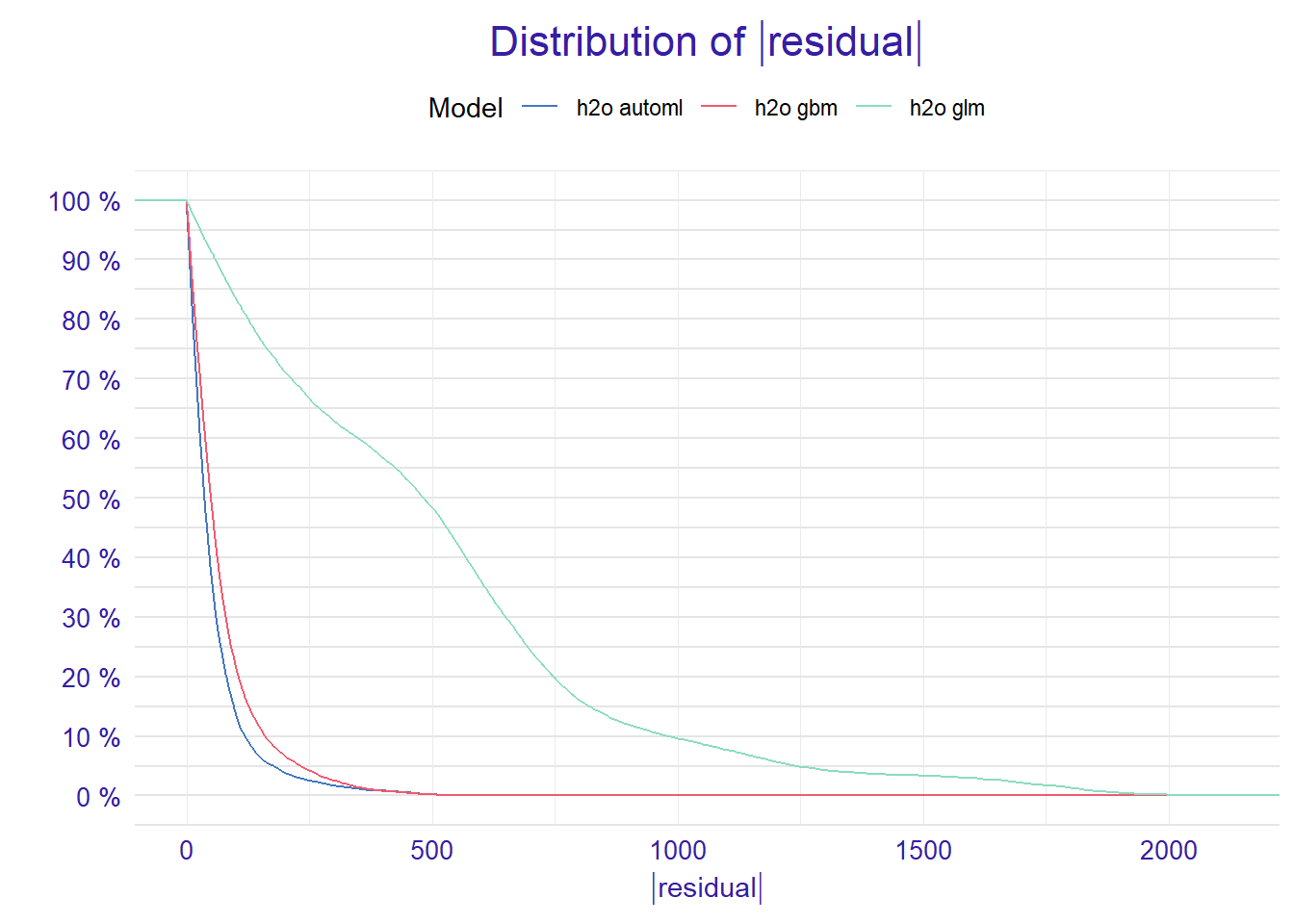We are also able to use the `plot()` function to get an alternative comparison of residuals. Setting the `geom = "boxplot"` parameter we can compare the distribution of residuals for selected models.

``plot(mp_h2o_glm, mp_h2o_gbm, mp_h2o_automl, geom = "boxplot")``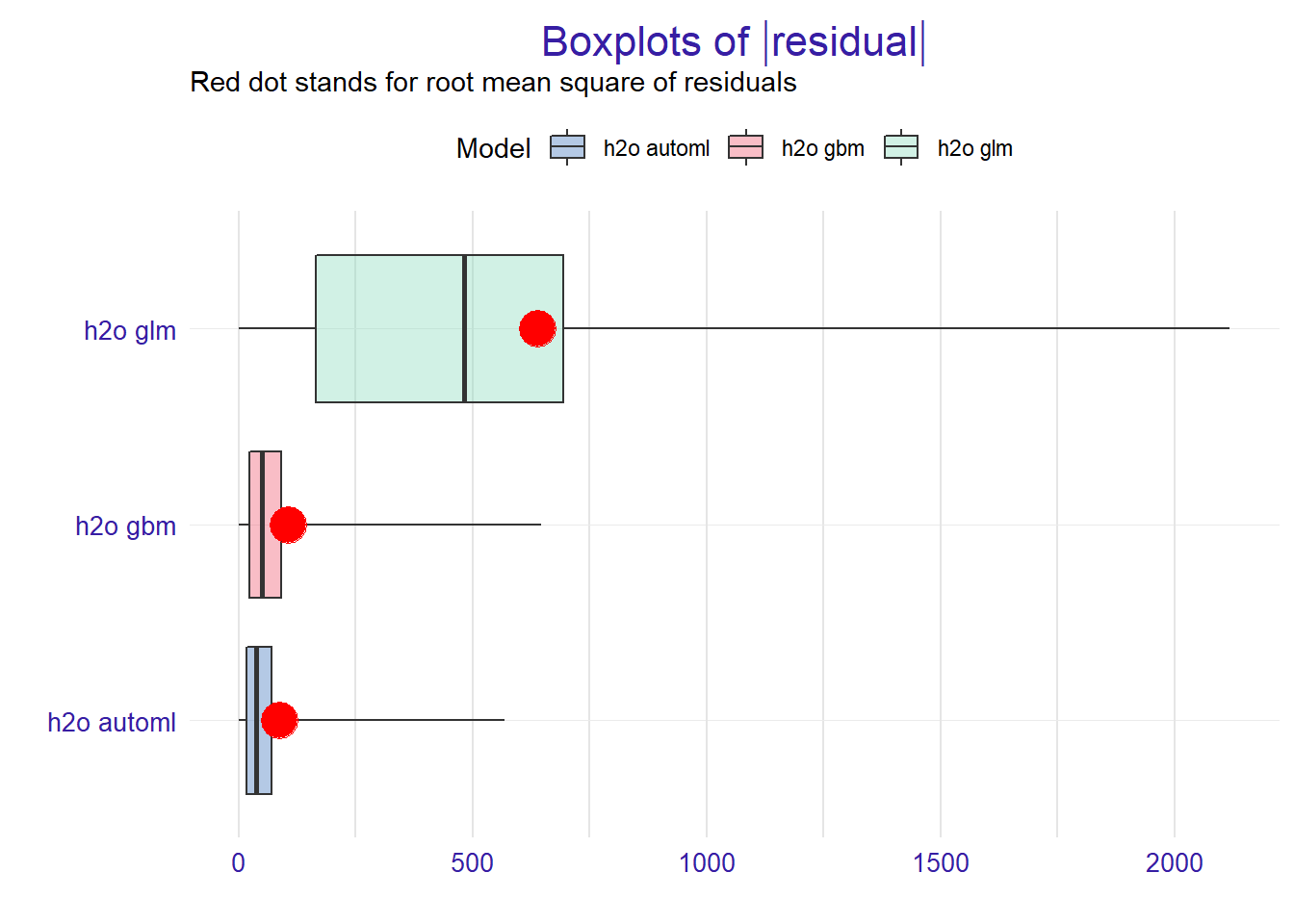# 6 Variable importance

Using he DALEX package we are able to better understand which variables are important.

Model agnostic variable importance is calculated by means of permutations. We simply substract the loss function calculated for validation dataset with permuted values for a single variable from the loss function calculated for validation dataset.

This method is implemented in the `variable_importance()` function.

``vi_h2o_glm <- variable_importance(explainer_h2o_glm)``
``````## Warning: Please note that 'variable_importance()' is now deprecated, it is better to use 'ingredients::feature_importance()' instead.
## Find examples and detailed introduction at: https://pbiecek.github.io/PM_VEE/featureImportance.html``````
``````vi_h2o_gbm <- variable_importance(explainer_h2o_gbm)
vi_h2o_automl <- variable_importance(explainer_h2o_automl)``````

We can compare all models using the generic `plot()` function.

``plot(vi_h2o_glm, vi_h2o_gbm, vi_h2o_automl)``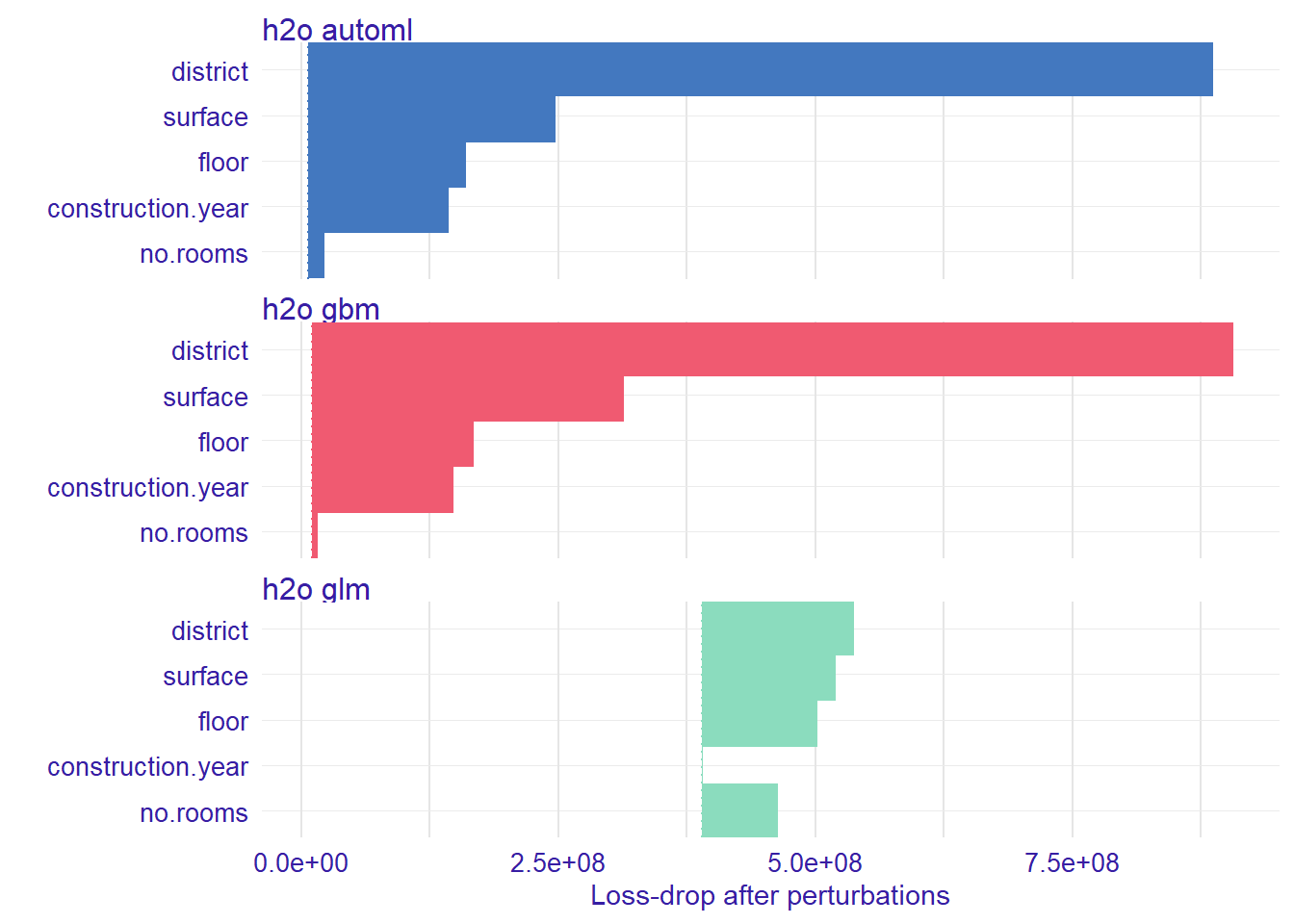Length of the interval coresponds to a variable importance. Longer interval means larger loss, so the variable is more important.

For better comparison of the models we can hook the variabe importance at 0 using the `type="difference"`.

``````vi_h2o_glm <- variable_importance(explainer_h2o_glm, type="difference")
vi_h2o_gbm <- variable_importance(explainer_h2o_gbm, type="difference")
vi_h2o_automl <- variable_importance(explainer_h2o_automl, type="difference")
plot(vi_h2o_glm, vi_h2o_gbm, vi_h2o_automl)``````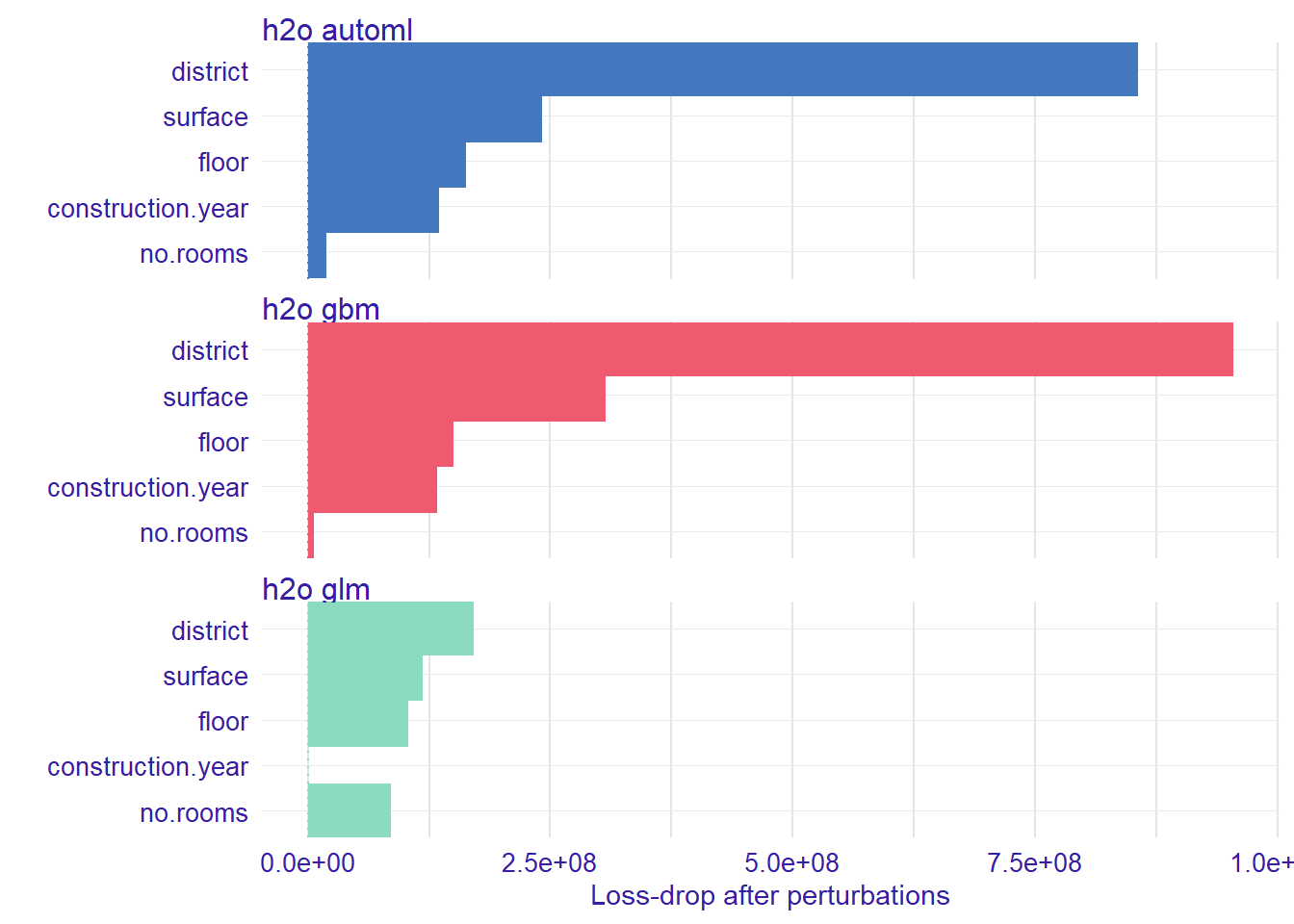# 7 Variable response

Explainers presented in this section are designed to better understand the relation between a variable and model output.

For more details of methods desribed in this section see Variable response section in DALEX docs.

## 7.1 Partial Dependence Plot

Partial Dependence Plots (PDP) are one of the most popular methods for exploration of the relation between a continuous variable and the model outcome.

Function `variable_response()` with the parameter `type = "pdp"` calls `pdp::partial()` function to calculate PDP response.

``pdp_h2o_glm <- variable_response(explainer_h2o_glm, variable = "construction.year")``
``````## Warning: Please note that 'variable_response()' is now deprecated, it is better to use 'ingredients::partial_dependency()' instead.
## Find examples and detailed introduction at: https://pbiecek.github.io/PM_VEE/partialDependenceProfiles.html``````
``````pdp_h2o_gbm <- variable_response(explainer_h2o_gbm, variable = "construction.year")
pdp_h2o_automl <- variable_response(explainer_h2o_automl, variable = "construction.year")

plot(pdp_h2o_glm, pdp_h2o_gbm, pdp_h2o_automl)``````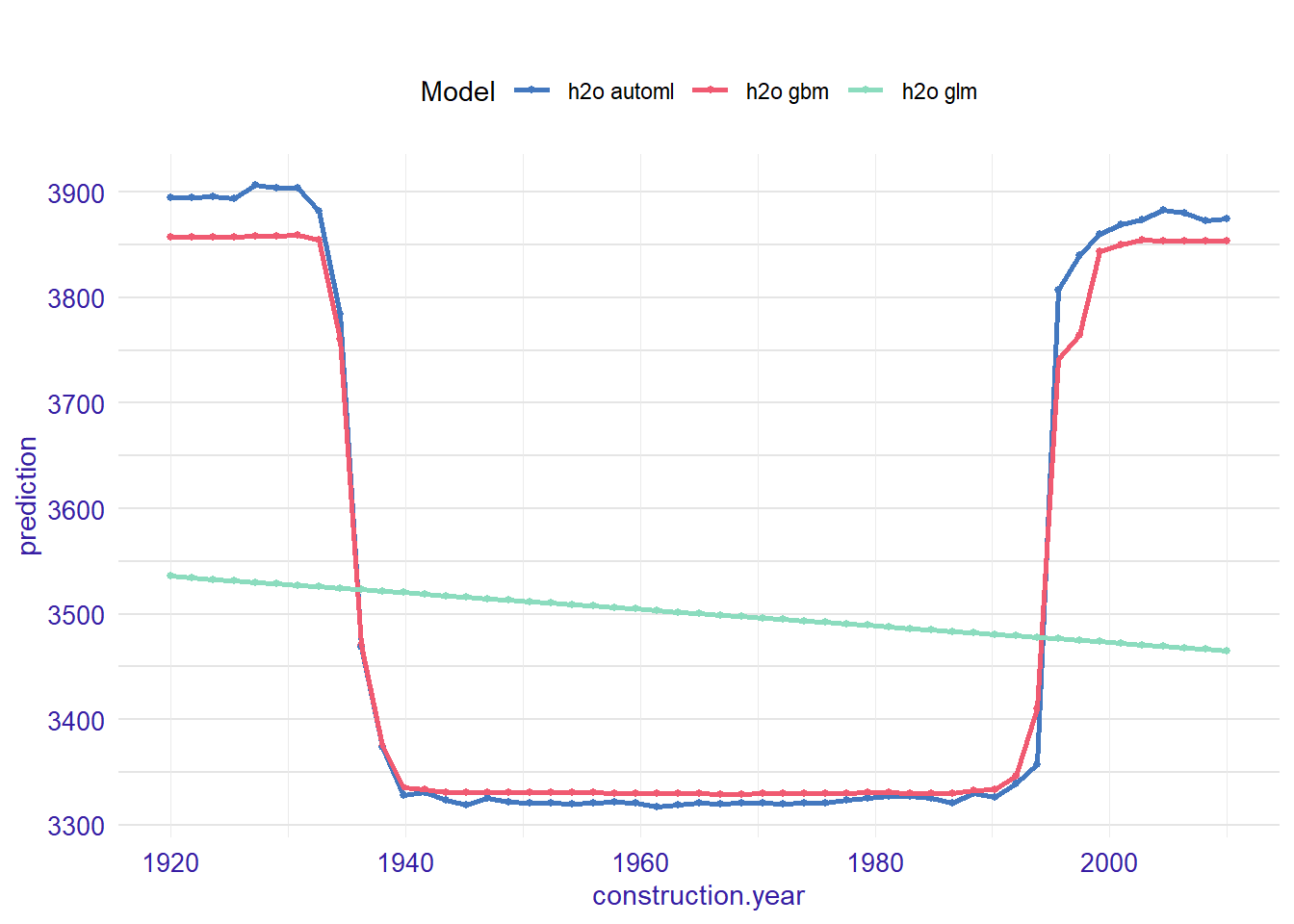## 7.2 Acumulated Local Effects plot

Acumulated Local Effects (ALE) plot is the extension of PDP, that is more suited for highly correlated variables.

Function `variable_response()` with the parameter `type = "ale"` calls `ALEPlot::ALEPlot()` function to calculate the ALE curve for the variable `construction.year`.

``ale_h2o_glm <- variable_response(explainer_h2o_glm, variable = "construction.year", type = "ale")``
``````## Warning: Please note that 'variable_response()' is now deprecated, it is better to use 'ingredients::accumulated_dependency()' instead.
## Find examples and detailed introduction at: https://pbiecek.github.io/PM_VEE/accumulatedLocalProfiles.html``````
``````ale_h2o_gbm <- variable_response(explainer_h2o_gbm, variable = "construction.year", type = "ale")
ale_h2o_automl <- variable_response(explainer_h2o_automl, variable = "construction.year", type = "ale")

plot(ale_h2o_glm, ale_h2o_gbm, ale_h2o_automl)``````